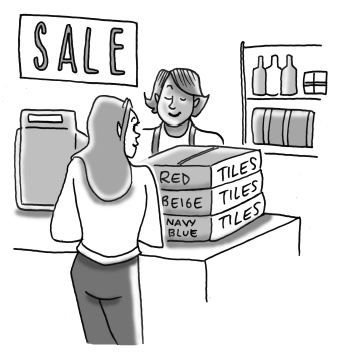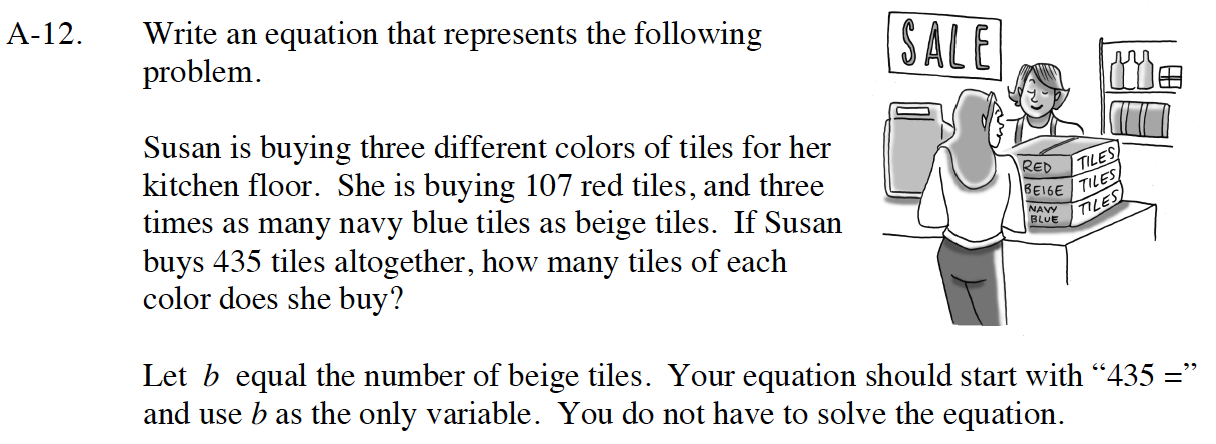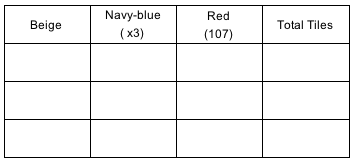Home > CC4 > Chapter A > Lesson A.1.1 > ProblemA-12

A-12.
1.Write an equation that represents the following problem.

2. Susan is buying three different colors of tiles for her kitchen floor. She is buying 107 red tiles, and three times as many navy blue tiles as beige tiles. If Susan buys 435 tiles altogether, how many tiles of each color does she buy?

3. Let b equal the number of beige tiles. Your equation should start with “435 =” and use b as the only variable. You do not have to solve the equation. Homework Help ✎Choose a number for the amount of beige tiles.Multiply the number of beige tiles by 3 to get the number of navy-blue tiles.

Add the beige, red, and navy-blue tiles together to get the total number of tiles Susan bought. Did your total come out to 435? If not, try another number for the beige.